2023年VeighNa小班特训营【机器学习CTA】、【套利价差交易】报名进行中，目前半数名额已经被报名锁定，感兴趣的同学请抓紧！内容大纲戳这里

### 增加同时持仓数量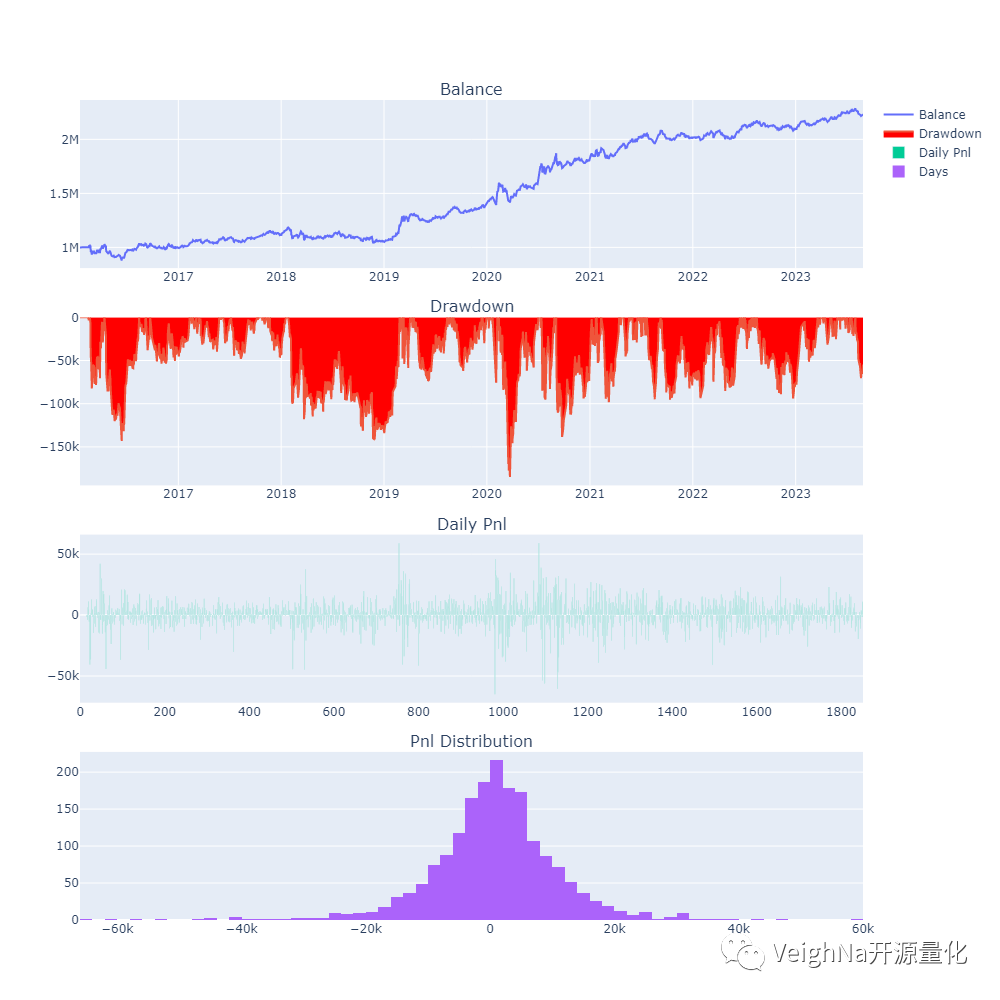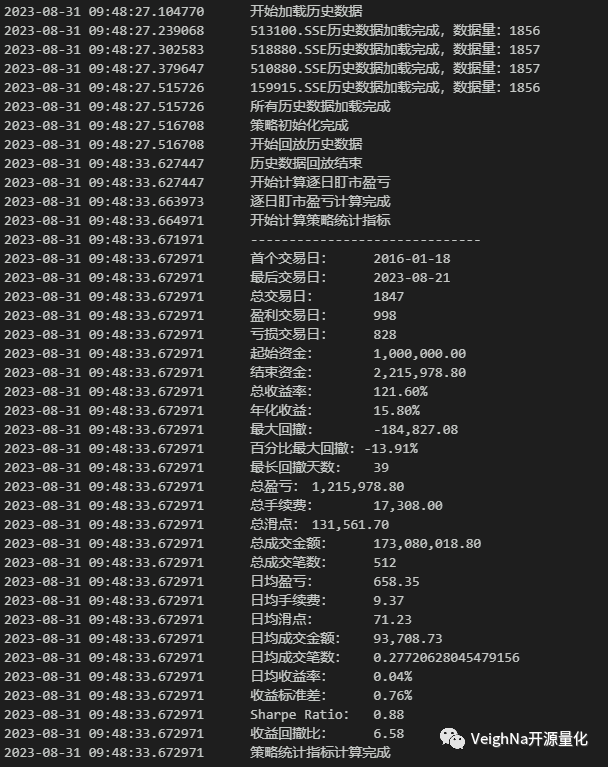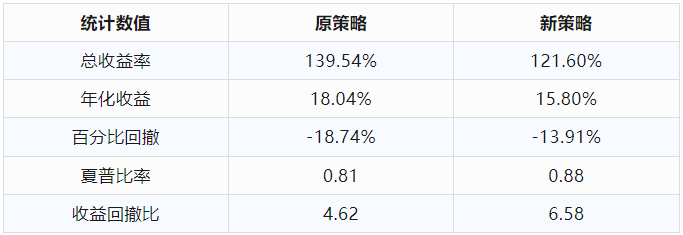### 策略代码实现

```class EtfRotationStrategy(StrategyTemplate):
"""ETF轮动策略"""

author: str = "CZL"

regression_window: int = 25         # 线性回归窗口
holding_size: int = 2               # 同时持仓数量
fixed_capital: int = 1_000_000      # 固定持仓市值

parameters = [
"regression_window",
"holding_size",
]

def on_init(self) -> None:
"""策略初始化"""
# 确保缓存数据足够回归计算
size: int = self.regression_window + 1

# 创建缓存持仓合约的列表
self.holding_symbols: list = []

# 创建每个合约的时序数据容器
self.ams: dict[str, ArrayManager] = {}

for vt_symbol in self.vt_symbols:
self.ams[vt_symbol] = ArrayManager(size)

self.write_log("策略初始化")
```

```def on_bars(self, bars: dict[str, BarData]) -> None:
"""K线切片推送"""
# 更新K线到时序容器
score_data: Dict[str, float] = {}

for vt_symbol, bar in bars.items():
am: ArrayManager = self.ams[vt_symbol]
am.update_bar(bar)

for vt_symbol, bar in bars.items():
am: ArrayManager = self.ams[vt_symbol]
if not am.inited:
return

# 对ETF进行打分
score_data[vt_symbol] = calculate_score(am.close[-self.regression_window:])

# 重置目标仓位
self.set_target(vt_symbol, 0)

# 对score进行排序，切片选出得分靠前的N个ETF
top_ranked = sorted(score_data, key=score_data.get, reverse=True)[:self.holding_size]

# 如果新一轮入选的ETF与目前持仓不一样，进行调仓
if set(top_ranked) != set(self.holding_symbols):
self.holding_symbols = top_ranked
for security in self.holding_symbols:
price: float = bars[security].close_price

# 每个入选的合约获得同样资金
volume: int = 100 * int((self.fixed_capital / self.holding_size) / (price * 100))
self.set_target(security, volume)

# 根据设置好的目标仓位进行交易
self.rebalance_portfolio(bars)

# 推送UI更新
self.put_event()
```

### 策略历史回测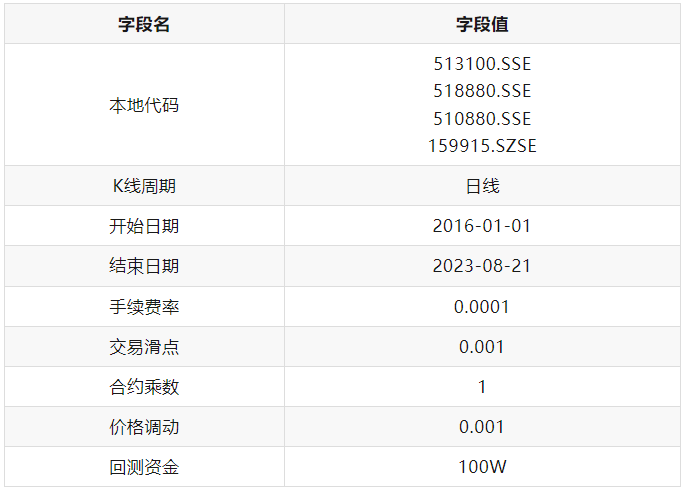### 策略参数优化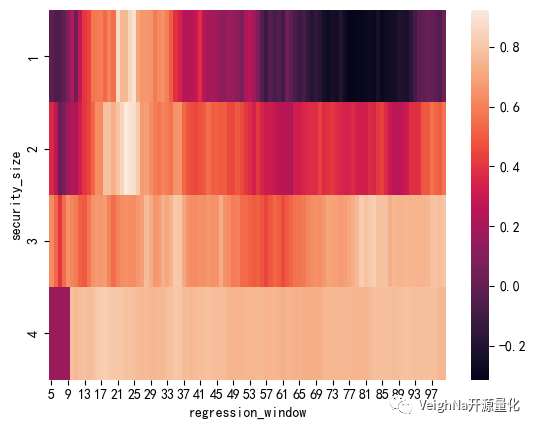```# 这里需要用到pandas和seaborn两个包
import pandas as pd
import seaborn as sns

# 先运行穷举优化，并将优化结果缓存
setting = OptimizationSetting()
setting.set_target("sharpe_ratio")
result = engine.run_optimization(setting)

# 创造一个空字典用于缓存
data = {}

# 遍历解析result中数据，并缓存进入data
for i in range(len(result)):
d.setdefault('holding_size', []).append(eval(result[i-1])['holding_size'])
d.setdefault('regression_window', []).append(eval(result[i-1])['regression_window'])
d.setdefault('sharpe_ratio', []).append(result[i-1])

# 将数据转化为DataFrame，并按照夏普比率的大小进行排序
sorted_result = (pd.DataFrame(data)
.sort_values('sharpe_ratio', ascending=False)
.reset_index(drop=True))

# 将数据格式转化为pivot table方便绘制热力图
pivoted_table = sorted_result.pivot("holding_size", "regression_window", "sharpe_ratio")

# 绘制热力图
sns.heatmap(pivoted_table)
```